# The base protonation constant K of azetidine (C2H NH) is 1.5 x 10 Calculate the pH...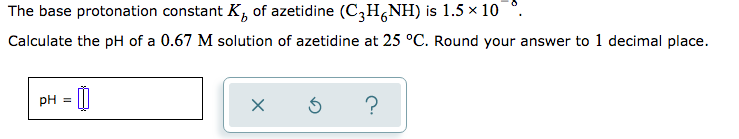The base protonation constant K of azetidine (C2H NH) is 1.5 x 10 Calculate the pH of a 0.67 M solution of azetidine at 25 °C. Round your answer to 1 decimal place. x ?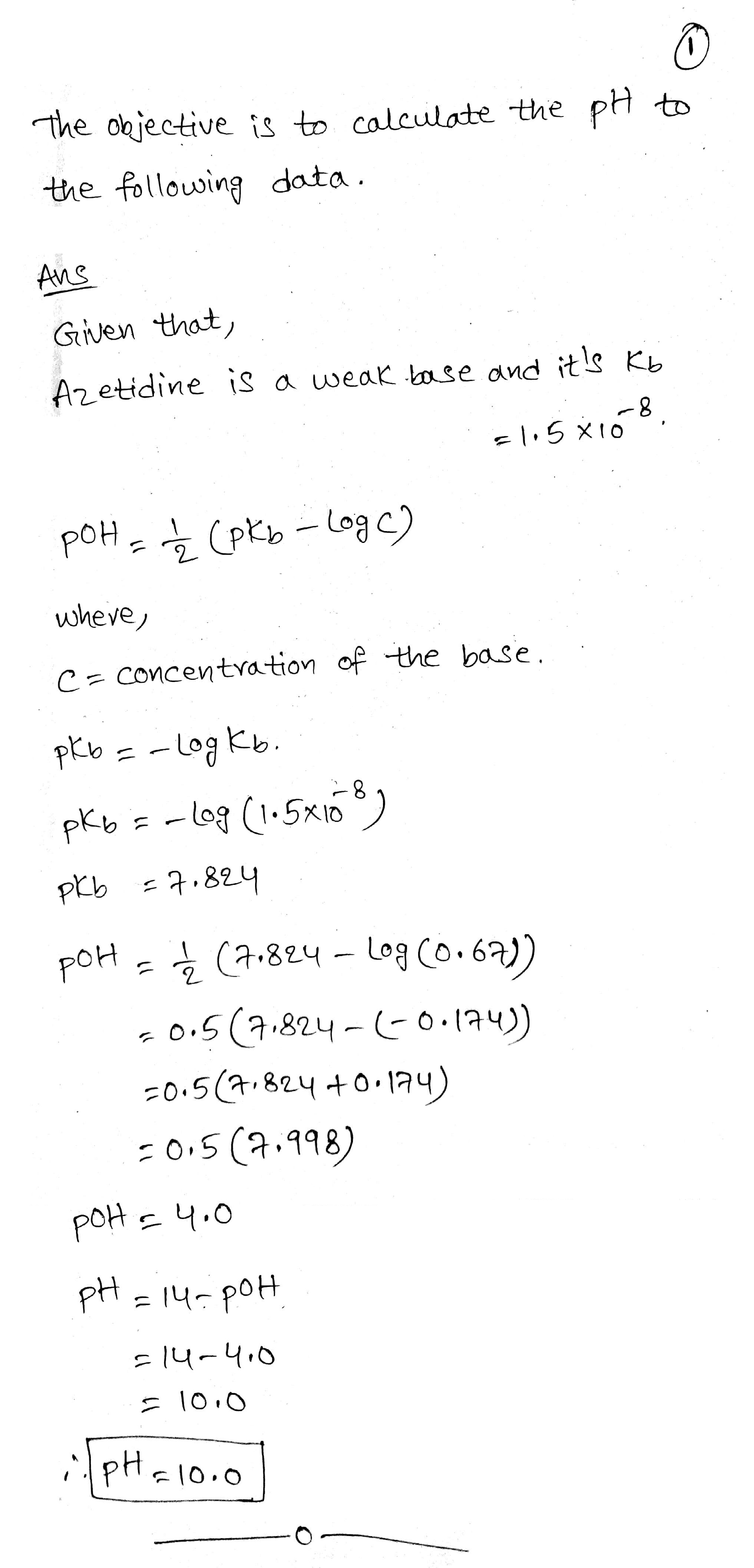#### Earn Coin

Coins can be redeemed for fabulous gifts.

Similar Homework Help Questions
• ### The base protonation constant K, of azetidine (C2H NH) is 1.5 x 10”. Calculate the pH of a 1.4 M solution of azetid...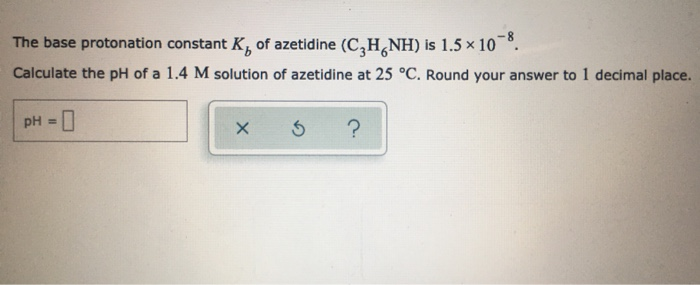The base protonation constant K, of azetidine (C2H NH) is 1.5 x 10”. Calculate the pH of a 1.4 M solution of azetidine at 25 °C. Round your answer to 1 decimal place. pH = 0 . I ?

• ### The base protonation constant K of allantoin (C4H.N,O,NH) is 9.12 x 10. Calculate the pH of...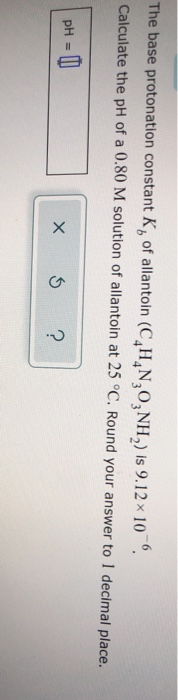The base protonation constant K of allantoin (C4H.N,O,NH) is 9.12 x 10. Calculate the pH of a 0.80 M solution of allantoin at 25 °C. Round your answer to 1 decimal place. pH = 0 x 3 ?

• ### The base protonation constant K, of azetidine (C,H NH) is 1.5x 108, Calculate the pH of...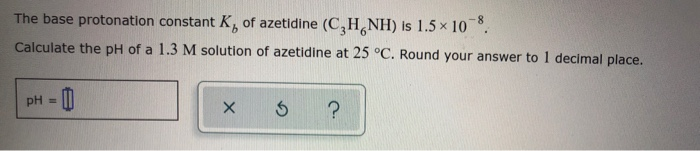The base protonation constant K, of azetidine (C,H NH) is 1.5x 108, Calculate the pH of a 1.3 M solution of azetidine at 25 °C. Round your answer to 1 decimal place. pH = 0) . 5 ?

• ### The base protonation constant K, of morpholine (C,H,ONH) is 2.14x 10 Calculate the pH of a...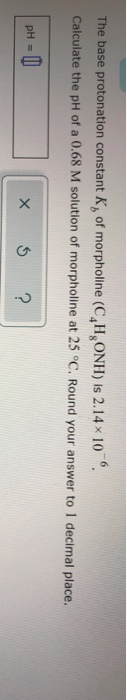The base protonation constant K, of morpholine (C,H,ONH) is 2.14x 10 Calculate the pH of a 0.68 M solution of morpholine at 25 °C. Round your answer to 1 decimal place. pH = 0 X 5 ?

• ### The base protonation constant K, of 1-H-imidazole (CHN) is 9.0x 10, Calculate the pH of a...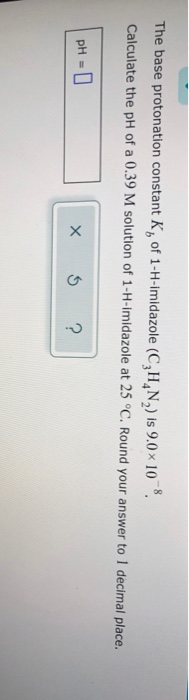The base protonation constant K, of 1-H-imidazole (CHN) is 9.0x 10, Calculate the pH of a 0.39 M solution of 1-H-Imidazole at 25 °C. Round your answer to 1 decimal place. pH = 0 XI ?

• ### The base protonation constant K, of lidocaine (C, H, NONH) is 1.15 10 . Calculate the...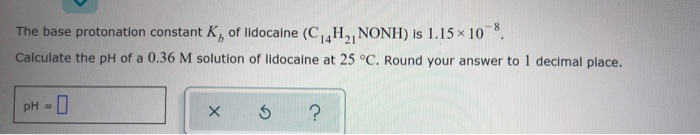The base protonation constant K, of lidocaine (C, H, NONH) is 1.15 10 . Calculate the pH of a 0.36 M solution of lidocaine at 25 °C. Round your answer to 1 decimal place. pH = 0 x 6 ?

• ### The base protonation constant Kb of lidocaine (C 14H2INONH) is 1.15 × 10 Calculate the pH...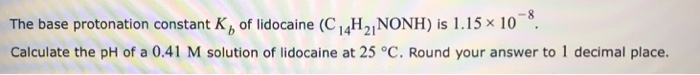The base protonation constant Kb of lidocaine (C 14H2INONH) is 1.15 × 10 Calculate the pH of a 0.41 M solution of lidocaine at 25 °C. Round your answer to 1 decimal place.

• ### The base protonation constant Kb of lidocane (C14H21NONH) is 1.5x10^-8. Calculate the pH of a 3.33...

The base protonation constant Kb of lidocane (C14H21NONH) is 1.5x10^-8. Calculate the pH of a 3.33 M solution of lidocane at 25 degrees Celcius. Round to 1 decimal place.

• ### Calculate the pH of a Weak Base Question The weak base dimethylamine, NH(CHs)2, has a molar...Calculate the pH of a Weak Base Question The weak base dimethylamine, NH(CHs)2, has a molar mass of 45.09 g/mol and a base-dissociation constant K, 5.4 x 10. What is the pH of an aqueous solution of dimethylamine that contains 2.04 g of dimethylamine in 0.100 L of solution? Use pK 14.0 for the ion-product of water. Round your answer to one decimal place. Provide your answer below pi-14.0 FEEDBACK MORE INSTRUCTION Content attribution

• ### 8) The base-ionization constant of ethylamine (C2H-NH2) is 6.4 x 10-4 at 25.0 °C. The [H*]...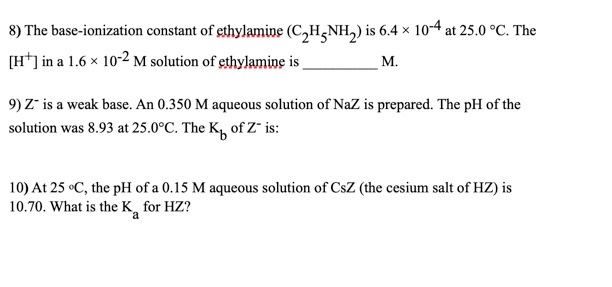8) The base-ionization constant of ethylamine (C2H-NH2) is 6.4 x 10-4 at 25.0 °C. The [H*] in a 1.6 x 10-2 M solution of ethylamine is M. 9) Z is a weak base. An 0.350 M aqueous solution of NaZ is prepared. The pH of the solution was 8.93 at 25.0°C. The Ky of Z" is: 10) At 25 C, the pH of a 0.15 M aqueous solution of Csz (the cesium salt of HZ) is 10.70. What is the...